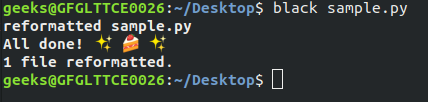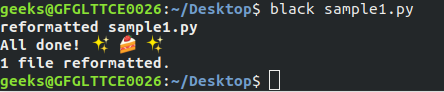# Python code formatting using Black

• Difficulty Level : Medium
• Last Updated : 05 Aug, 2021

Writing well-formatted code is very important, small programs are easy to understand but as programs get complex they get harder and harder to understand. At some point, you can’t even understand the code written by you. To avoid this, it is needed to write code in a readable format. Here Black comes into play, Black ensures code quality.

#### What is Black?

Linters such as pycodestyle or flake8 show whether your code is according to PEP8 format, which is the official Python style guide. But the problem is that it gives a burden to the developers to fix this formatting style. Here Black comes into play not only does it report format errors but also fixes them.To quote the project README:

Black is the uncompromising Python code formatter. By using it, you agree to cede control over minutiae of hand-formatting. In return, Black gives you speed, determinism, and freedom from pycodestyle nagging about formatting. You will save time and mental energy for more important matters.

Note: Black can be easily integrated with many editors such as Vim, Emacs, VSCode, Atom or a version control system like GIT.

#### Installing and using Black :

Black requires Python 3.6.0+ with pip installed :

`\$ pip install black`

It is very simple to use black. Run the below command in the terminal.

`\$ black [file or directory]`

This will reformat your code using the Black codestyle.
Example 1:Let’s create an unformatted file name “sample.py” and we want to format it using black. Below is the implementation.

## Python3

 `def` `is_unique(``               ``s``               ``):``    ``s ``=` `list``(s``                ``)``    ``s.sort()`  `    ``for` `i ``in` `range``(``len``(s) ``-` `1``):``        ``if` `s[i] ``=``=` `s[i ``+` `1``]:``            ``return` `0``    ``else``:``        ``return` `1`  `if` `__name__ ``=``=` `"__main__"``:``    ``print``(``          ``is_unique(``input``())``         ``)`

After creating this file run the below command.Output file:

## Python3

 `def` `is_unique(s):``    ``s ``=` `list``(s)``    ``s.sort()` `    ``for` `i ``in` `range``(``len``(s) ``-` `1``):``        ``if` `s[i] ``=``=` `s[i ``+` `1``]:``            ``return` `0``    ``else``:``        ``return` `1`  `if` `__name__ ``=``=` `"__main__"``:``    ``print``(is_unique(``input``()))`

In the above example, both are the same piece code but after using black it is formatted and thus easy to understand.
Example 2: Let’s create another file “sample1.py” containing the following code.

## Python3

 `def` `function(name, default``=``None``, ``*``args, variable``=``"1123"``, a, b, c, employee, office, d, e, f, ``*``*``kwargs):``    ``"""This is function is created to demonstrate black"""`  `string ``=` `'GeeksforGeeks'` `j ``=` `[``1``,`` ``2``,`` ``3``]`

After writing the above command again in the terminal.Output file:

## Python3

 `def` `function(``    ``name,``    ``default``=``None``,``    ``*``args,``    ``variable``=``"1123"``,``    ``a,``    ``b,``    ``c,``    ``employee,``    ``office,``    ``d,``    ``e,``    ``f,``    ``*``*``kwargs``):``    ``"""This is function is created to demonstrate black"""`  `string ``=` `"GeeksforGeeks"` `j ``=` `[``1``, ``2``, ``3``]`

My Personal Notes arrow_drop_up